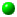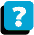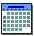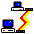Module 4.4 Notes "Comparing Multiple Samples of Numerical Data: One Factor"

 Index to Module 4 Notes4.1 Comparing 2 Independent Samples of Numerical Data4.2 Comparing 2 Related Samples of Numerical Data4.3 Comparing 2 Samples Using a Nonparametric Test4.4 Comparing Multiple Samples: One Factor4.5 Comparing Multiple Samples: Two Factors4.6 Comparing Multiple Samples: A Nonparametric Test

In Module Notes 4.1 we discussed methods designed to compare means of two independent samples to determine if the means of the populations from which they were drawn are equal or not. In Module Notes 4.2 we presented a method for comparing means of two related samples to determine if the means of the populations from which they were drawn are equal or not. Finally, Module Notes 4.3 provided a nonparametric method of comparing two independent samples to determine of the medians of the populations from which they were drawn are equal or not, when the assumption of normal population and equal variances could not be met.

We are now going to expand the concept presented in Module Notes 4.1 to compare means of multiple samples (beyond two) to determine if the means of the populations from which they were drawn are equal or not. Suppose we were interested in comparing means of three samples to determine if the means of the populations from which they were drawn are equal or not. The hypotheses statements are:

H0: MeanA = MeanB = MeanC
Ha: At least two Means are not equal

The parametric technique we use to conduct the analysis is called Analysis of Variance (ANOVA). The title Analysis of Variance may imply that we are going to compare variances, and not means. Actually we are going to compare means, but the way we do it is to compare the difference or variation between the means of the three groups to the variation within the groups. If the variation between the means is greater than the variation within the groups, we go with the alternative hypothesis.

Formulas for computing between group variation and within group variation are available in the references at the end of this set of module notes. We are going to let Excel do the computations. The important thing to remember is that the between group variation simply measures how the sample means compare to the grand average of all of the data. Does at least one group have a sample mean much greater or much less than the grand average of all the data? If "yes" (meaning the difference between the means is significantly greater than the within group variation), then we reject the null hypothesis and conclude at least two of the means are not equal. The within group variation I am referring to measures how the individual observations within a group differ from their group sample mean.

When the sample means are far from each other relative to the within group variation, "something" is going on. In the miles per gallon scenario, the brands of gasoline really have different properties that shifts the average mpg of one or more groups away from other another group or groups. Simply put, the average mpg is significantly different between the groups. When the sample means are close together relative to the within group variation, we say that any difference between sample means is due to chance - nothing "special" is going on to cause the means to be different.

You may recall that we already studied an ANOVA table - we did that in regression. In that case, we compared the variation attributed to the regression model to the variation that was unexplained (the error or residual). We are going to use the ANOVA table again, this time comparing between group variation to within group variation.

The Situation

I will return to the miles per gallon study introduced in Module Notes 4.1. Here is the data:

Worksheet 4.4.1
 Brand A Brand B Brand C 20 20 20 20 20.5 26 19 18.5 23 16 20 24 15 19 23 17 19 25 14 18 23

The objective is to compare the mean mpg of group A, with group B with group C to determine if there is or is not a difference between the means. The hypotheses statements are presented in the introduction above. We are going to use the F statistic because we are comparing the ratio of two variances (between group variation to within group variation).

The tool we use in Excel is a Data Analysis add in. So, we select Tools from the Standard Toolbar, Data Analysis from the pulldown menu, then ANOVA Single Factor. Then respond to the dialog box questions. Note that my data is in columns, and I defaulted to an alpha of 0.05. Single Factor means we are studying one factor that may result in the means being different - that factor is the type or brand of gasoline put into the 7 cars in each of the three groups. The results (output) of the ANOVA analysis are shown below:

Worksheet 4.4.2
 Anova: Single Factor SUMMARY Groups Count Sum Average Variance Brand A 7 121 17.28571429 5.904761905 Brand B 7 135 19.28571 0.821429 Brand C 7 164 23.42857143 3.619047619 ANOVA Source of Variation SS df MS F P-value F crit Between Groups 137.4286 2 68.71429 19.92635 2.73237E-05 3.554561 Within Groups 62.07143 18 3.448413 Total 199.5 20

The first thing to note in the ANOVA output are the descriptive statistics which include the means and the variances of the three samples. These are followed by the ANOVA Table which has the variation separated as Between Groups (variation between the three means and the grand mean) and Within Groups. Since the p-value of 2.732E-05 is less than the alpha value of 0.05, we reject the null hypothesis and conclude that there is a difference between the means. Thus, "something" special is going on - the brands of gas do have a significant differential effect on the mpg performance.

Now that we have some statistics, we can look at the between and within group variation with numbers. Note that Group A's sample mean is 17.3, and Group C's sample mean is 23.4. The difference between these two groups is 6.1 mpg, a measure of the between group variation. The variation within Group A is 2.4 and within group C it's 1.9. Using the larger of the two, we see that the difference between the groups of 6.1 is much larger than the largest of the two variations within the groups, hence we rejected the null hypothesis... "something" is going on to cause the difference other than chance alone. In the actual computation, the ANOVA technique computes and uses a pooled variance as the measure of variation within the group, so the above was done simply to illustrate the concept.

Note carefully the alternative hypothesis simply says there is a difference between the means somewhere, but at this point, we don't know where that is - we suspect it's between A and C as demonstrated above. To find the answer to the question, "which pairs of means are different," we do a post hoc test (post hoc meaning a follow-on test after the ANOVA). We would only do this when we reject the null hypothesis, since whenever we fail to reject the null hypothesis in ANOVA, we are saying there is no difference between the means.

The Bonferroni Multiple Comparisons Procedure
The Bonferroni Procedure (Sincich, Business Statistics by Example) uses information from the ANOVA table, and a t-Test statistic, so it can be done in Excel. There are other post hoc tests, such as the Tukey Cramer procedure (Levine, 1999), but these require special tables not available in Excel. I believe the Bonferroni has higher utility to the manager interested in using the statistical capability of Excel.

The first thing to do is determine how many pairs of means we want to test for differences. Since there are three groups, we can do a maximum of three tests: compare the mean of A with B, the mean of A with C and the mean of B with C.

The second thing to do is determine the difference between the sample means:

Eq. 4.4.1: | MeanA - MeanB | = | 17.3 - 19.3 | = 2
Eq. 4.4.2: | MeanA - MeanC | = | 17.3 - 23.4 | = 6.1
Eq. 4.4.3: | MeanB - MeanC | = | 19.3 - 23.4 | = 4.1
where | | means absolute value.

The third task is to compute the Bonferroni critical difference statistic, B. This statistic becomes the threshold value for comparison. Any difference between sample means (such as those shown in Equations 4.4.1 - 4.4.3) greater than B is a statistically significant difference - those two means are not equal. Any difference between sample means less than B is not a significant difference - those two means are equal.

Equation 4.4.4 provides the formula for B:

Eq. 4.4.4: B = t (alpha/2g)* Sq Root (MS Within Groups) *
Sq Root /[(1/n1) + (1/n2)]

where: g = number of pair wise comparisons being made. Note, if there are three groups, the maximum number of comparisons is 3*(3-1)/2 = 3; if there are five groups, the maximum is 5*(5-1)/2 = 10; n1 and n2 are the sample sizes of the two groups being compared.

The t(alpha/2g) can be obtained from Excel. Let's let alpha be 0.05, g is 3, so alpha/2*3 would be 0.00833. To get a t-score for 0.00833 with 18 degrees of freedom associated with the Within Group variation (see ANOVA Table in Worksheet 4.4.2 above) we use the =TINV(alpha, degrees of freedom) function in Excel. In an active cell in an Excel Worksheet, select Insert on the Standard Toolbar, Function from the pulldown menu, Statistical, TINV, and enter 0.00833 for alpha and 18 for degrees of freedom. The function should look like this: =TINV(0.00833, 18) which gives a t score of 2.963.

The MS Within Groups is the Mean Square Within Groups obtained from the ANOVA table (3.4484). Now to put it all together:

Eq. 4.4.5: B = 2.963 * Sq Root(3.4484) * Sq Root [(1/7) + (1/7)]
B = 2.941

The Bonferroni Critical statistic is 2.941. Any pair wise difference between sample means greater than 2.941 is a statistically significant difference. Equation 4.4.2 and 4.4.3 show that the difference between means of groups A and C and B and C are greater than 2.941; therefore those pairs of means are significantly different. On the other hand, the difference between the means of A and B is only 2 (Eq. 4.4.1); that is not a significant difference, but rather only due to chance.

ANOVA Assumptions

There are three assumptions needed to employ the one-way or one factor ANOVA model.

The first assumption is randomness and independence. To avoid bias, it is important the the data be gathered and placed in the multiple groups in a random, objective manner so that the observations are independent of each other. Thus, the one factor ANOVA, also called the One Factor Completely Randomized Design, does not apply to related samples (such as the same car being given Brand of Gas A, then B, then C).

The second assumption is the normality assumption: the sampled groups are drawn from normally distributed populations, just as in the case of the t-Test for independent samples. The ANOVA procedure works fairly well as long as there are not extreme departures from normality, especially if the sample sizes are large. If there are departures from normality, as examined by looking at descriptive statistics for the samples, and if the sample sizes are small (less than 30), the nonparametric technique presented in Module Notes 4.4.6 is the preferred alternative.

The last assumption is called homogeneity of variance. This assumptions requires that the variances of the multiple groups be equal. This is necessary because the variances of each group are pooled to create the single within group variation used in comparison to the between group variation in the ANOVA procedure. If the sample sizes are the same within each group, inferences made may not be seriously affected. However, if the sample sizes are not the same, and the variances are unequal, there is a serious effect on the inferences made.

When both the normality and homogeneity of variance assumptions are violated, it is best to revert to the nonparametric procedure presented in Module Notes 4.6.

References:

Anderson, D., Sweeney, D., & Williams, T. (2001). Contemporary Business Statistics with Microsoft Excel. Cincinnati, OH: South-Western, Chapter 10 (Sections 10.4 - 10.5).

Levine, D., Berenson, M. & Stephan, D. (1999). Statistics for Managers Using Microsoft Excel (2nd. ed.). Upper Saddle River, NJ: Prentice-Hall, Chapter 10.

Mason, R., Lind, D. & Marchal, W. (1999). Statistical Techniques in Business and Economics (10th. ed.). Boston: Irwin McGraw Hill, Chapter 11.

Sincich, T. (1992). Business Statistics by Example, (4th. ed.). New York: Dellen.About the CourseModule ScheduleWebBoard